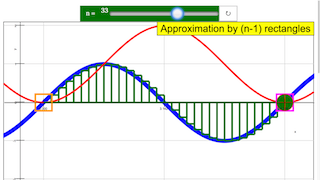Intro Page

Definite integral

This simulation demonstrates definite integration of the sine function by the simple algorithm of summing approximative rectangles. The red curve shows the sine function itself.

The definite integral is to be calculated between initial point x1 (blue) an end point x2 (magenta). A first slider defines x1 ; x2 can be drawn with the mouse.

The red curve is the analytic antiderivative for an initial value x1: y = cos(x) - cos (x1).

A second slider n defines the number n-1 of subinterval into which x2 - x1 is divided for the approximation. In each subinterval the approximative amplitude is assumed to be equal to its initial value. The sum of the area of all rectangles is shown by a green point at x2 .

Reset defines 1 < x < 4 and n-1 = 9.

Experiments

E1: Start with the default setting: x1 = 0; n = 10.

Draw the magenta end point and observe the deviation of the rectangle sum (green point) from the analytic solution.

E2: Understand why the deviation is negative for x < π/2, and why it becomes zero at x = π . Reflect how summing mistakes can compensate for periodic functions.

E3: Increase the number of intervals with the slider and observe how the deviation changes and disappears in the limit.

E4: Change the initial point and consider why and how this shifts the analytic solution.

E5: Choose a large integration interval and consider why the rectangles lie below or above certain parts of the function.

Translations

Code Language Translator RunSoftware Requirements

SoftwareRequirements

 Android iOS Windows MacOS with best with Chrome Chrome Chrome Chrome support full-screen? Yes. Chrome/Opera No. Firefox/ Samsung Internet Not yet Yes Yes cannot work on some mobile browser that don't understand JavaScript such as..... cannot work on Internet Explorer 9 and below

CreditsDieter Roess - WEH-Foundation; Fremont Teng; Loo Kang Wee

[text]

Definite Integral JavaScript Simulation Applet HTML5

Instructions

N Slider

Toggling the n slider will toggle the number of boxes used for approximation.

(n=49)

(n=6)

Draggable Boxes

Drag the orange/magenta boxes to set the points/coordinates on the curve.

Toggling Full Screen

Double click anywhere on the screen to toggle full screen.

Reset Button

Resets the simulation.

Research

[text]

[text]

[text]

Testimonials (0)

There are no testimonials available for viewing. Login to deploy the article and be the first to submit your review!